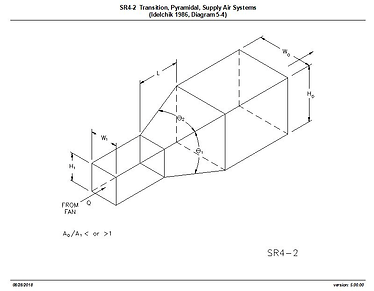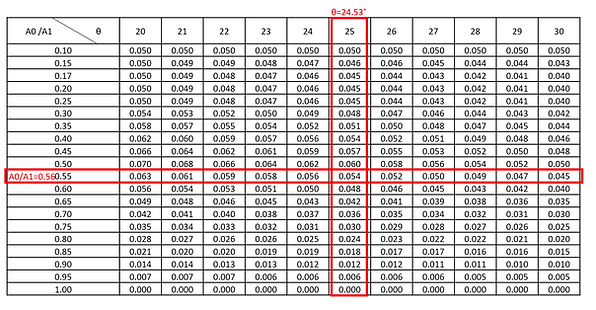top of page

Duct Transition Pressure Drop Calculator

According to the Ashrae fitting (SR4-2), the transition loss is based on a few parameters, namely

1. The length of transition, L

2. Pre-transition duct Width, W & Height, H (Contributes to Area A1)

3. Post-transition duct Width, W & Height, H (Contributes to Area A0)

4. Airflow, QBelow are the steps for the calculation of the losses based on a simple example above:

1. From W, H, and L, the angle θ for both the sides and and the top (θ1 and θ2) can be computed as shown below. Take the bigger value between the 2 angles (θ1 and θ2) as θ, i.e.

θ = MAX(θ1, θ2) = 24.53 deg2. Then, compute the ratio of Duct Cross Sectional Area,

A0/A1 = (300x300)/(400x400) = 0.753. From the Ashrae table, determine the Coefficient of Loss, C can be determined with information computed from Step 1 and 2 above.Where no exact value of theta and A0/A1 can be referenced in the fitting loss table, Interpolation using “Forecast” function in excel may be helpful to determine the Coefficient of Loss. From the interpolated data shown below, we see that the coefficient of loss, C = 0.054.4. Compute the Velocity Pressure, VP post-transition (for exhaust, pre-transition)

5. Compute Pressure Loss, ΔP byThe method for computing the transition loss for an exhaust or return system is also similar to the above, with the exception that the Ashrae fitting ER4-2 is referred instead of SR4-2 for supply. For both Supply and Return, the method of computing the loss is shown in the excel files attached below.

Exhaust Transition Loss Calculator ER4-2 (Excel)

Supply Transition Loss Calculator SR4-2 (Excel)bottom of page